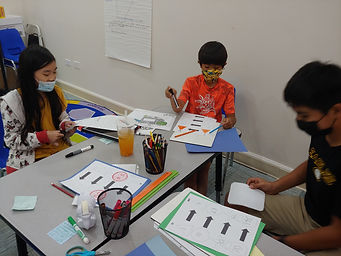Ms. Mari

Target 1​

Lesson Type:

New

Algebra

:

Function

Use input and output data to determine a function equation.

1:

Define a function as a rule that gives one output to each input.

2:

Use a function equation to fill out a function table.

7th

Vocabulary:

FUNCTION, INPUT, OUTPUT

Activities:

- Given an input and output, determined many rules for the function (as many as possible in three minutes).

- Given several inputs and outputs, determined one rule for the function.

- Created a function machine with their own rules and shared four different inputs and outputs using the rule.Home Exploration

Guiding Questions:Absent Students:

Target 2

:

Vocabulary:

Activities:Home Exploration

Guiding Questions:Target 3

:

Vocabulary:

Activities:Home Exploration